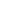SEARCH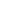BROWSEBégout, Pascal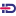and Ildefonso Diaz, Jesus(2023) Finite time extinction for a critically damped Schrödinger equation with a sublinear nonlinearity. Advances in Differential Equations, vol. 28 (N° 3-4). pp. 311-340. (In Press)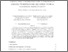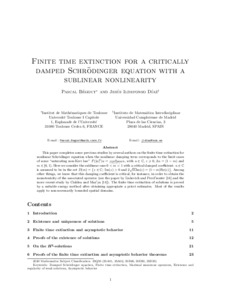Preview Text Download (493kB) | Preview

## Abstract

This paper completes some previous studies by several authors on the finite time extinction for nonlinear Schrödinger equation when the nonlinear damping term corresponds to the limit cases of some "saturating non-Kerr law" $F(|u|^2)u=\frac{a}{\varepsilon+(|u|^2)^\alpha}u,$ with $a\in\mathbb{C},$ $\varepsilon\geqslant0,$ $2\alpha=(1-m)$ and $m\in[0,1).$ Here we consider the sublinear case $0<m<1$ with a critical damped coefficient: $a\in\mathbb{C}$ is assumed to be in the set $D(m)=\big\{z\in\mathbb{C}; \; \mathrm{Im}(z)>0 \text{ and } 2\sqrt m\mathrm{Im}(z)=(1-m)\mathrm{Re}(z)\big\}.$ Among other things, we know that this damping coefficient is critical, for instance, in order to obtain the monotonicity of the associated operator (see the paper by Liskevich and Perel'muter  and the more recent study by Cialdea and Maz'ya ). The finite time extinction of solutions is proved by a suitable energy method after obtaining appropiate a priori estimates. Most of the results apply to non-necessarily bounded spatial domains.

Item Type: Article English 2023 Yes Athens, OH Damped Schrödinger equation, Finite time extinction, Maximal monotone operators, Existence and regularity of weak solutions, Asymptotic behavior Équations de Schrödinger amortie, Extinction en temps fini, Opérateurs maximaux monotones, Existence et régularité des solutions faibles, Comportement asymptotique G- MATHEMATIQUES Institut de mathématiques de Toulouse UT1 07 Nov 2022 14:20 07 Nov 2022 14:20 https://publications.ut-capitole.fr/id/eprint/46338